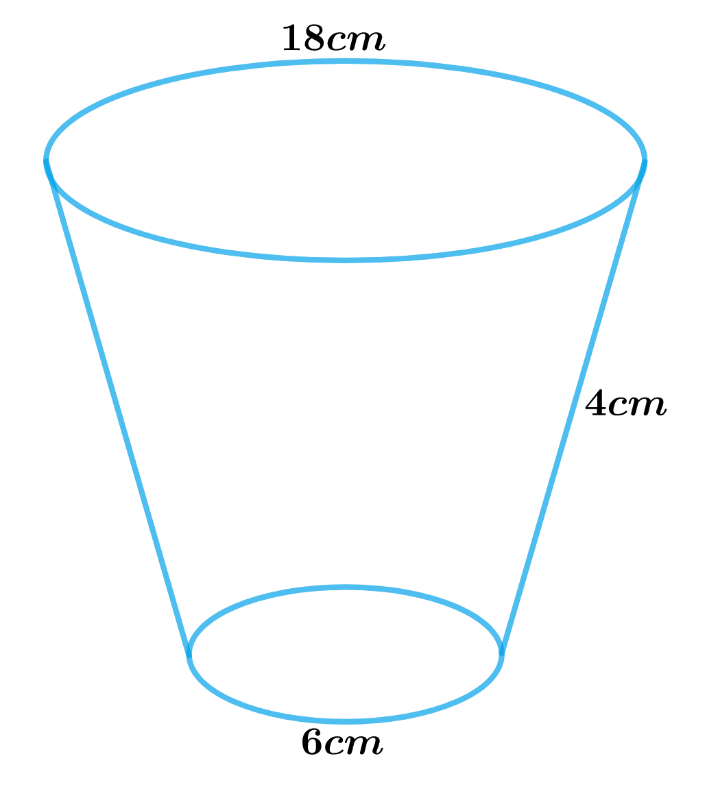# Ex.13.4 Q2 Surface Areas and Volumes Solution - NCERT Maths Class 10

Go back to  'Ex.13.4'

## Question

The slant height of a frustum of a cone is $$4\,\rm{cm}$$ and the perimeters (circumference) of its circular ends are $$18 \,\rm{cm}$$ and $$6\, \rm{cm.}$$ Find the curved surface area of the frustum.

Video Solution
Surface Areas And Volumes
Ex 13.4 | Question 2

## Text Solution

What is Known?

Slant height of a frustum of a cone is $$4\,\rm{cm}$$ and the circumference of its circular ends are $$18\, \rm{cm}$$ and $$6 \,\rm{cm.}$$

What is unknown?

The curved surface area of the frustum.

Reasoning:

Draw a figure to visualize the shape better

Using the circumferences of the circular ends of the frustum to find the radii of its circular ends

Circumference of the circle $$= 2\pi r$$

where $$r$$ is the radius of the circle.

We will find the CSA of the frustum by using formula;

CSA of frustum of a cone $$= \pi \left( {{r_1} + {r_2}} \right)l$$

where $$r1, r2$$ and $$l$$ are the radii and slant height of the frustum of the cone respectively.Steps:

Slant height of frustum of a cone, $$l = 4 \rm cm$$

Circumference of the larger circular end, $${C_1} = 18 \rm cm$$

Radius of the larger circular end,\begin{align} {r_1} = \frac{{{C_1}}}{{2\pi }} = \frac{{18 \rm cm}}{{2\pi }} = \frac{9}{\pi } \rm cm\end{align}

Circumference of the smaller circular end, $${C_2} = 6 \rm cm$$

Radius of the smaller circular end,\begin{align} {r_2} = \frac{{{C_2}}}{{2\pi }} = \frac{{6 \rm cm}}{{2\pi }} = \frac{3}{\pi } \rm cm\end{align}

CSA of frustum of a cone $$= \pi \left( {{r_1} + {r_2}} \right)l$$

\begin{align}&= \pi \left( {\frac{9}{\pi } \rm cm + \frac{3}{\pi } \rm cm} \right) \times 4 \rm cm\\&= \pi \times \frac{{12}}{\pi } \rm cm \times 4cm\\&= 48 \rm c{m^2}\end{align}

The curved surface area of the frustum is $$48 \;\rm cm^2.$$

Learn from the best math teachers and top your exams

• Live one on one classroom and doubt clearing
• Practice worksheets in and after class for conceptual clarity
• Personalized curriculum to keep up with school